Question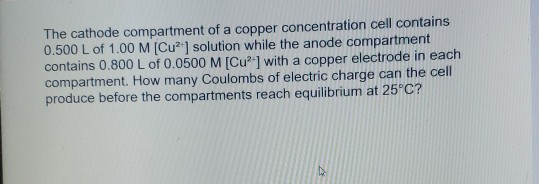We need at least 10 more requests to produce the answer.

0 / 10 have requested this problem solution

The more requests, the faster the answer.

All students who have requested the answer will be notified once they are available.

#### Earn Coins

Coins can be redeemed for fabulous gifts.

Similar Homework Help Questions
• ### The cathode compartment of a copper concentration cell contains 0.500 L of 1.00 M [Cu2+] solution...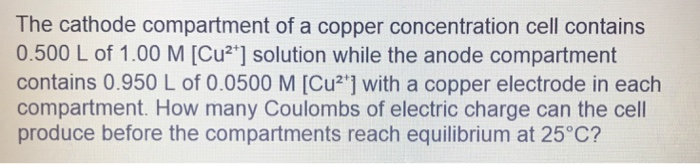The cathode compartment of a copper concentration cell contains 0.500 L of 1.00 M [Cu2+] solution while the anode compartment contains 0.950 L of 0.0500 M [Cu2"] with a copper electrode in each compartment. How many Coulombs of electric charge can the cell produce before the compartments reach equilibrium at 25°C?

• ### The cathode compartment of a copper concentration cell contains 0.500 L of 1.00 M (Cu*] solution...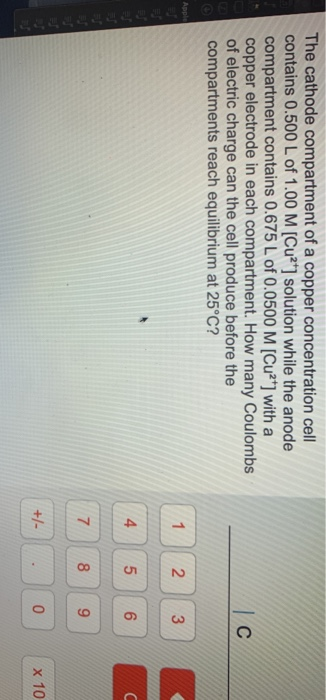The cathode compartment of a copper concentration cell contains 0.500 L of 1.00 M (Cu*] solution while the anode compartment contains 0.675 L of 0.0500 M [Cu2+] with a copper electrode in each compartment. How many Coulombs of electric charge can the cell produce before the compartments reach equilibrium at 25°C? с Apple 1 2 3 4 5 U 6 7 8 9 +1 0 x 10

• ### 3 ques plz help thank you The cathode compartment of a copper concentration cell contains 0.500...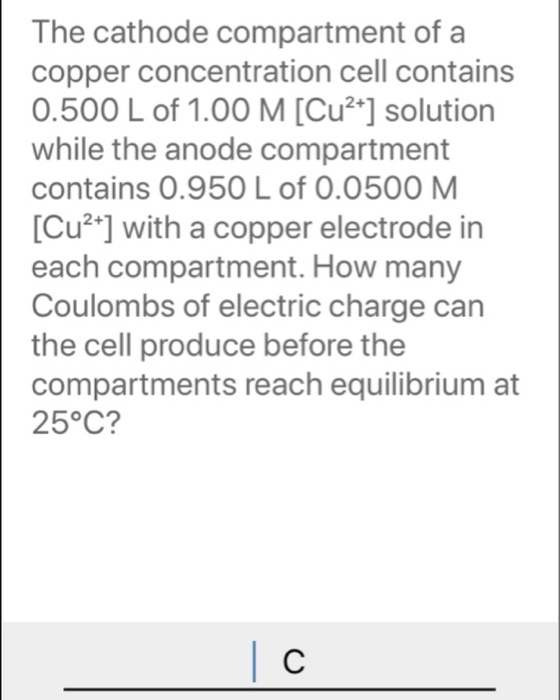3 ques plz help thank you The cathode compartment of a copper concentration cell contains 0.500 L of 1.00 M [Cu2+] solution while the anode compartment contains 0.950 L of 0.0500 M. [Cu2+] with a copper electrode in each compartment. How many Coulombs of electric charge can the cell produce before the compartments reach equilibrium at 25°C? | C Calculate the atomic mass of the magnesium-27 nucleus in amu (to three decimal places) assuming that the mass of a nucleon...

• ### need help plz 3 ques What is the correct systematic name for the compound shown here?...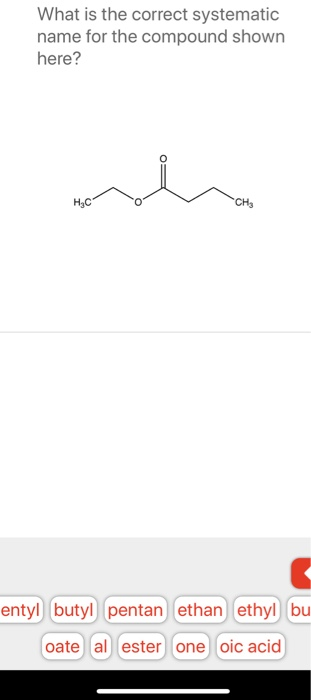need help plz 3 ques What is the correct systematic name for the compound shown here? HEC CHE entyl butyl pentan ethan ethyl bu oate al ester one oic acid The cathode compartment of a copper concentration cell contains 0.500 L of 1.00 M [Cu2+] solution while the anode compartment contains 0.950 L of 0.0500 M. [Cu2+] with a copper electrode in each compartment. How many Coulombs of electric charge can the cell produce before the compartments reach equilibrium at...

• ### *A copper, Cu(s), electrode is immersed in a solution that is 1.00 M in ammonia, NH3, and 1.00 M in tetraamminecopper(II), [Cu(NH3)4]2+. If a standard hydrogen electrode is used as the cathode, the ce...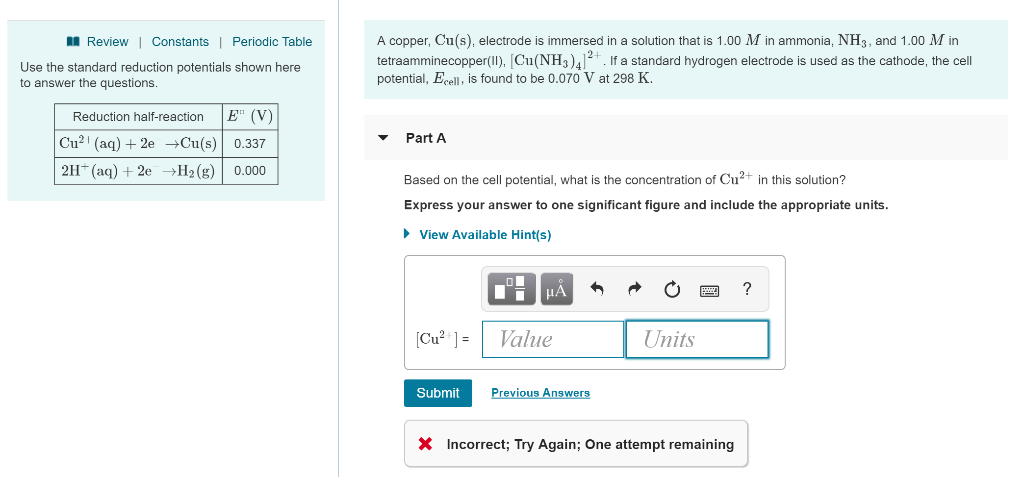*A copper, Cu(s), electrode is immersed in a solution that is 1.00 M in ammonia, NH3, and 1.00 M in tetraamminecopper(II), [Cu(NH3)4]2+. If a standard hydrogen electrode is used as the cathode, the cell potential, Ecell, is found to be 0.070 V at 298 K. A copper, Cu(s), electrode is immersed in a solution that is 1.00 M in ammonia, NH3, and 1.00 M in tetraamminecopper(II), [NH. If a standard hydrogen electrode is used as the cathode, the cell potential,...

• ### 14.__/15 pts) In a concentration cell, a voltage can be created when one compartment has a...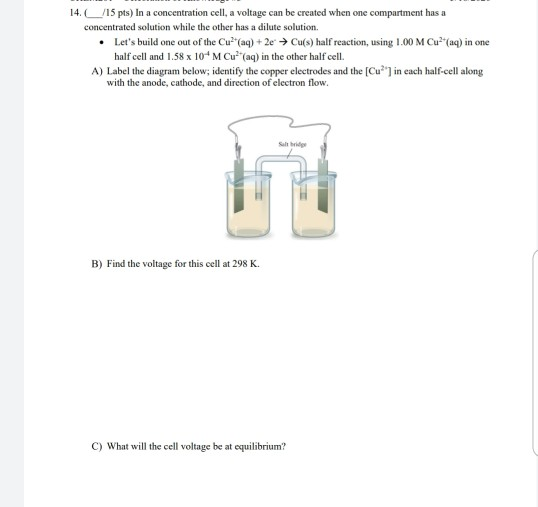14.__/15 pts) In a concentration cell, a voltage can be created when one compartment has a concentrated solution while the other has a dilute solution. • Let's build one out of the Cu?"(aq) + 2e → Cu(s) half reaction, using 1.00 M Cu?"(aq) in one half cell and 1.58 x 10 M Cu?"(aq) in the other half cell. A) Label the diagram below; identify the copper electrodes and the [Cu?") in each half-cell along with the anode, cathode, and direction...

• ### salt bridge ME Cr(s) electrode Cu(s) electrode 1.0 M Cr3+ (aq) 1.0 M Cu2+ (aq) A...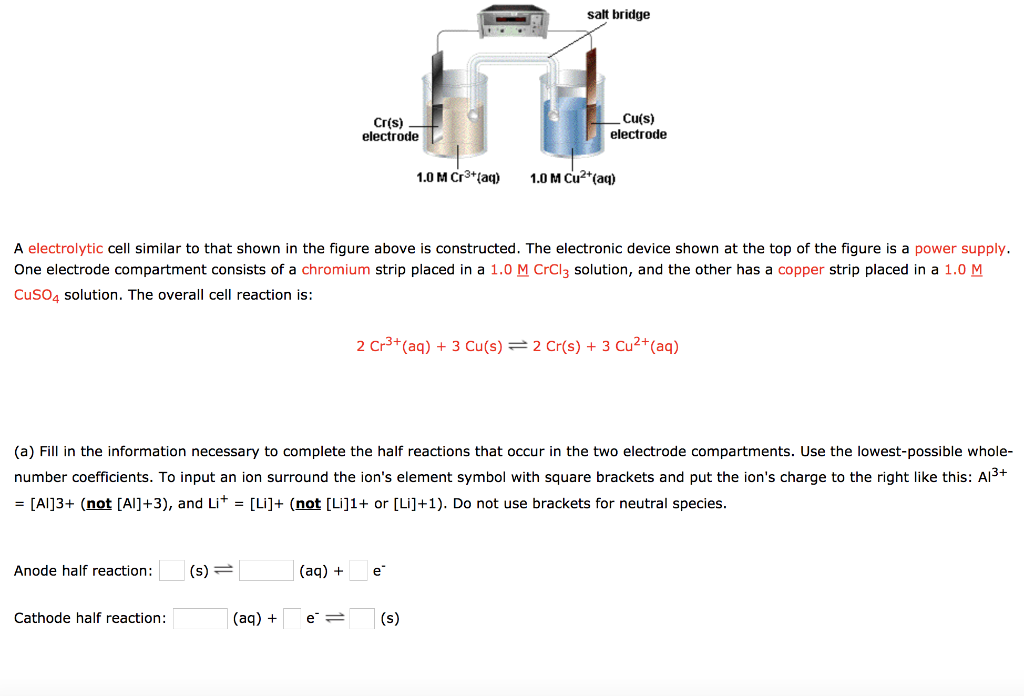salt bridge ME Cr(s) electrode Cu(s) electrode 1.0 M Cr3+ (aq) 1.0 M Cu2+ (aq) A electrolytic cell similar to that shown in the figure above is constructed. The electronic device shown at the top of the figure is a power supply. One electrode compartment consists of a chromium strip placed in a 1.0 M CrCl3 solution, and the other has a copper strip placed in a 1.0 M CuSO4 solution. The overall cell reaction is: 2 Cr3+ (aq) +...

• ### A copper, Cu(s), electrode is immersed in a solution that is 1.00 M in ammonia, NH3,...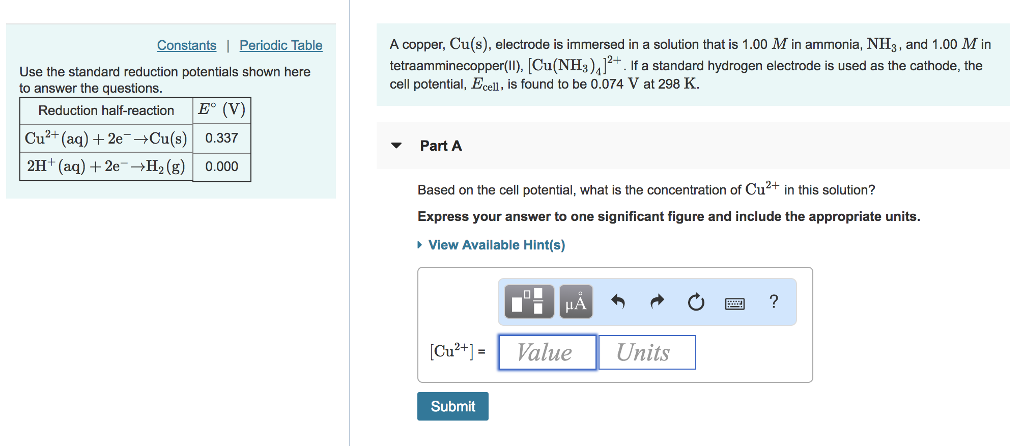A copper, Cu(s), electrode is immersed in a solution that is 1.00 M in ammonia, NH3, and 1.00 M in tetraamminecopper(I), [Cu(NH). If a standard hydrogen electrode is used as the cathode, the cell potential, Ecell, is found to be 0.074 V at 298 K Constants Periodic Table Use the standard reduction potentials shown here to answer the questions Reduction half-reaction E (V) Cu2+ (aq) 2e Cu(s) 0.337 2H + (aq) + 2e →H, (g) | 0.000 ▼ Part A...

• ### In a copper-zinc voltaic cell, one half-cell consists of a ZnZn electrode inserted in a solution...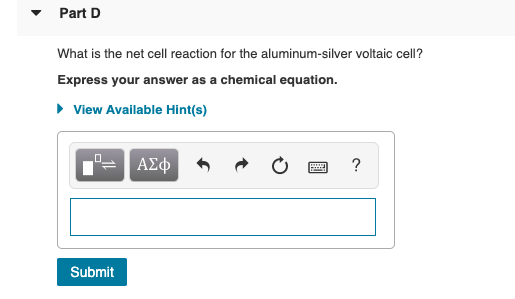In a copper-zinc voltaic cell, one half-cell consists of a ZnZn electrode inserted in a solution of zinc sulfate and the other half-cell consists of a CuCu electrode inserted in a copper sulfate solution. These two half-cells are separated by a salt bridge. At the zinc electrode (anode), ZnZn metal undergoes oxidation by losing two electrons and enters the solution as Zn2+Zn2+ ions. The oxidation half-cell reaction that takes place at the anode is Zn(s)→Zn2+(aq)+2e−Zn(s)→Zn2+(aq)+2e− The CuCu ions undergo reduction...

• ### And a copper electrode with 0.500 M Cu?' as the second half cell Cu2+ (aq) +...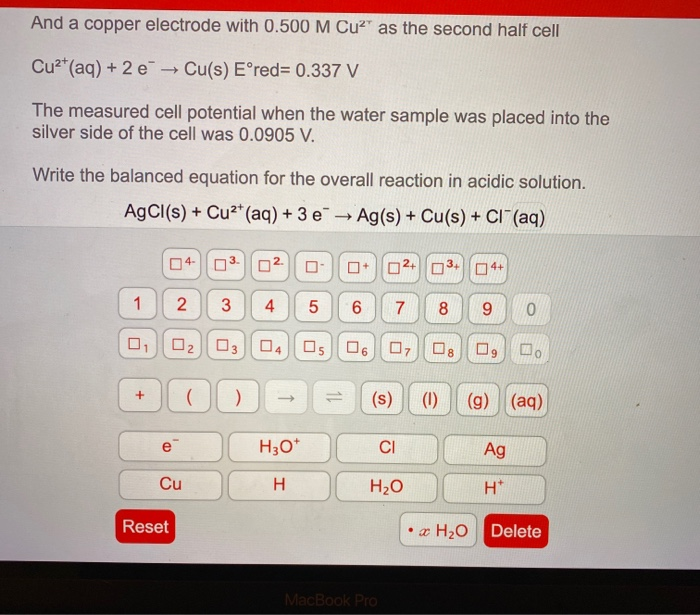And a copper electrode with 0.500 M Cu?' as the second half cell Cu2+ (aq) + 2 e Cu(s) Eºred= 0.337 V The measured cell potential when the water sample was placed into the silver side of the cell was 0.0905 V. Write the balanced equation for the overall reaction in acidic solution. AgCl(s) + Cu2+ (aq) + 3 e → Ag(s) + Cu(s) + CI+ (aq) 04- 03. 02. + 2 O 4+ 1 2 3 4 5 6...